Chapter 8 Class 12 Application of Integrals
Serial order wise

## The bridge connects two hills 100 feet apart. The arch on the bridge is in a parabolic form. The highest point on the bridge is 10 feet above the road at the middle of the bridge as seen in the figure.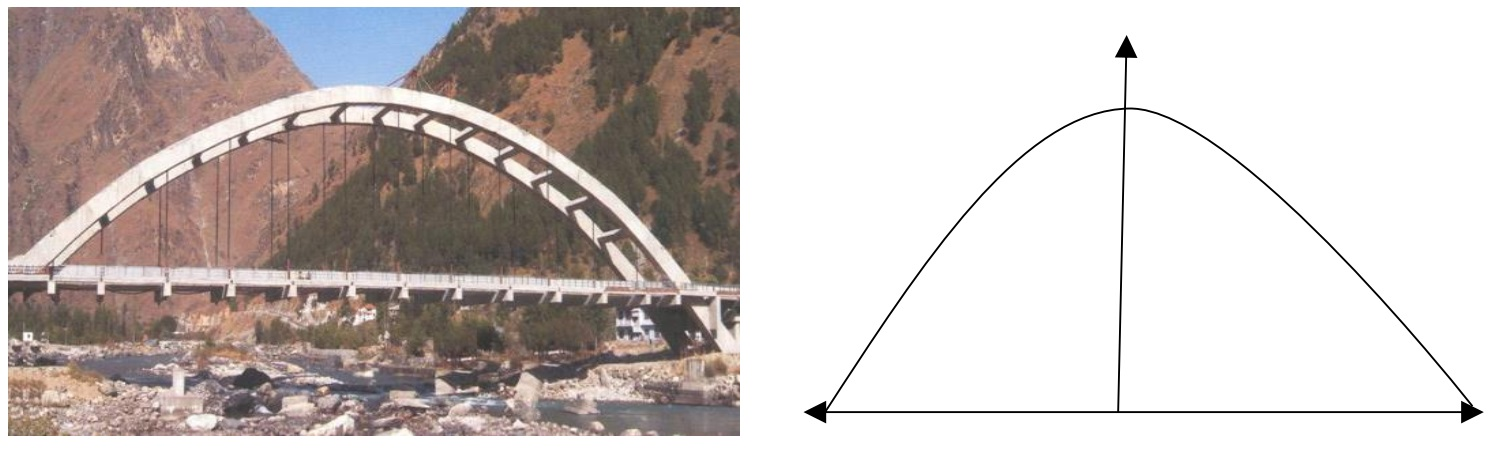googletag.cmd.push(function() { googletag.display('div-gpt-ad-1669298377854-0'); }); Based on the information given above, answer the following questions: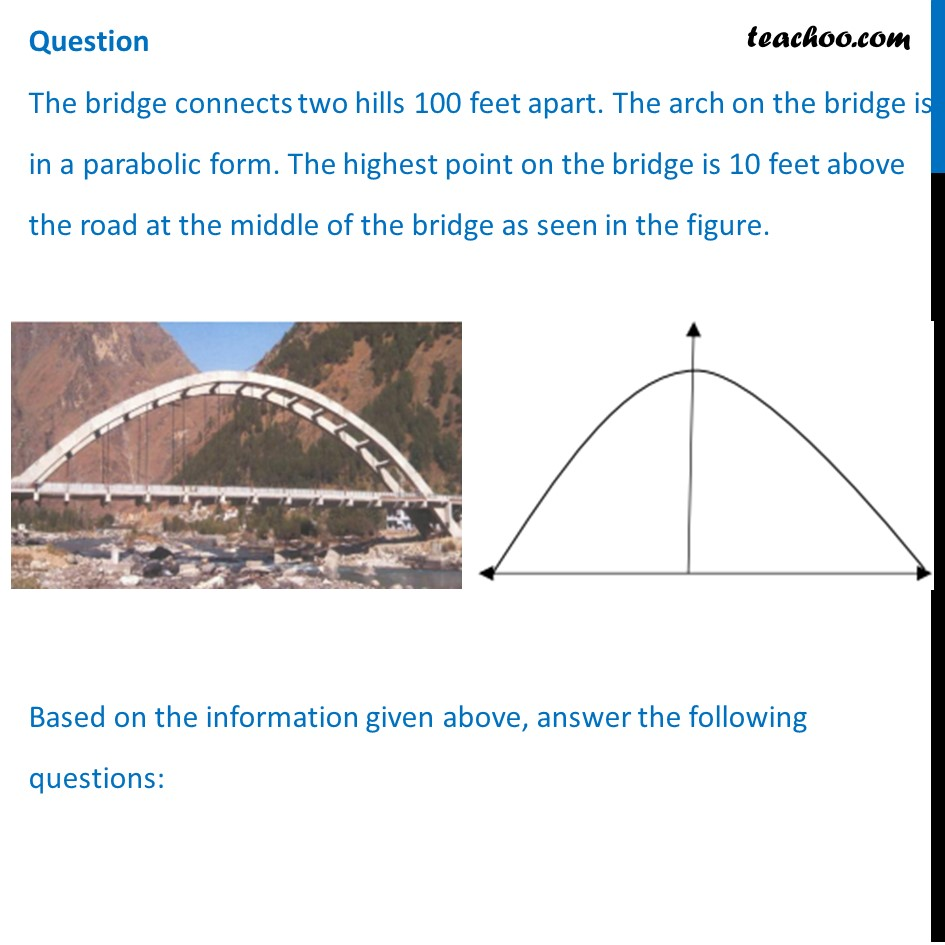## (d) y 2 = 250 𝑦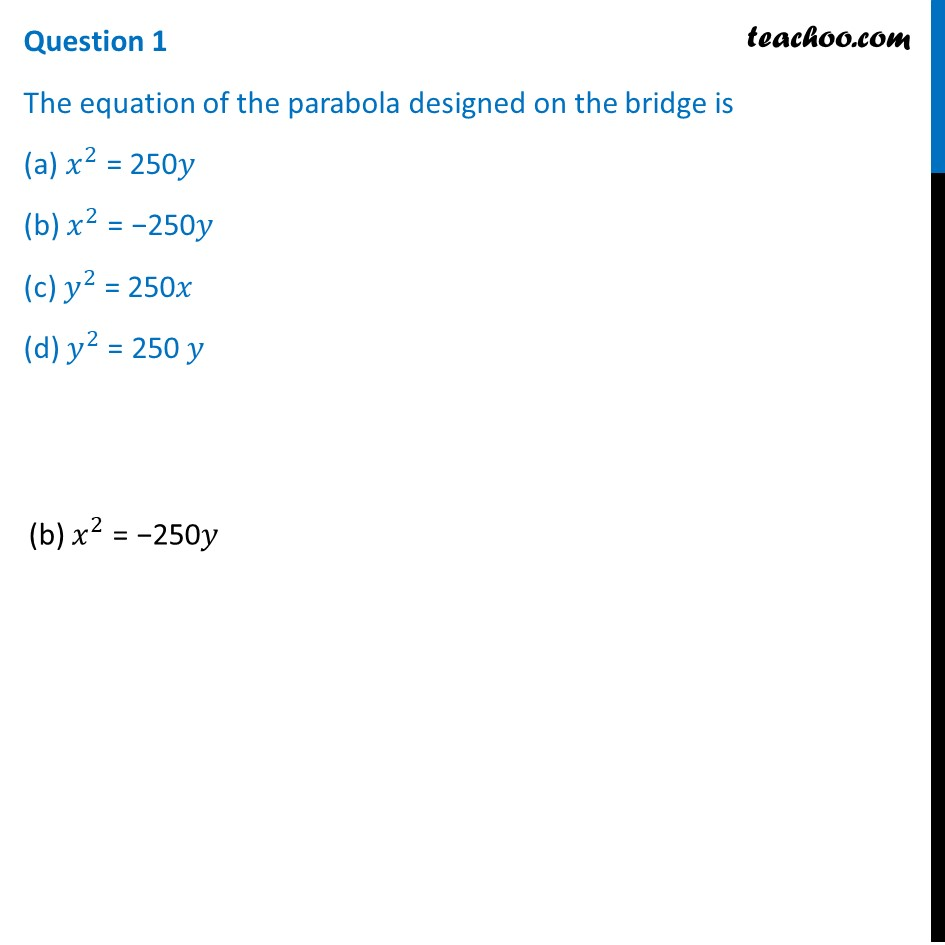## (d) 0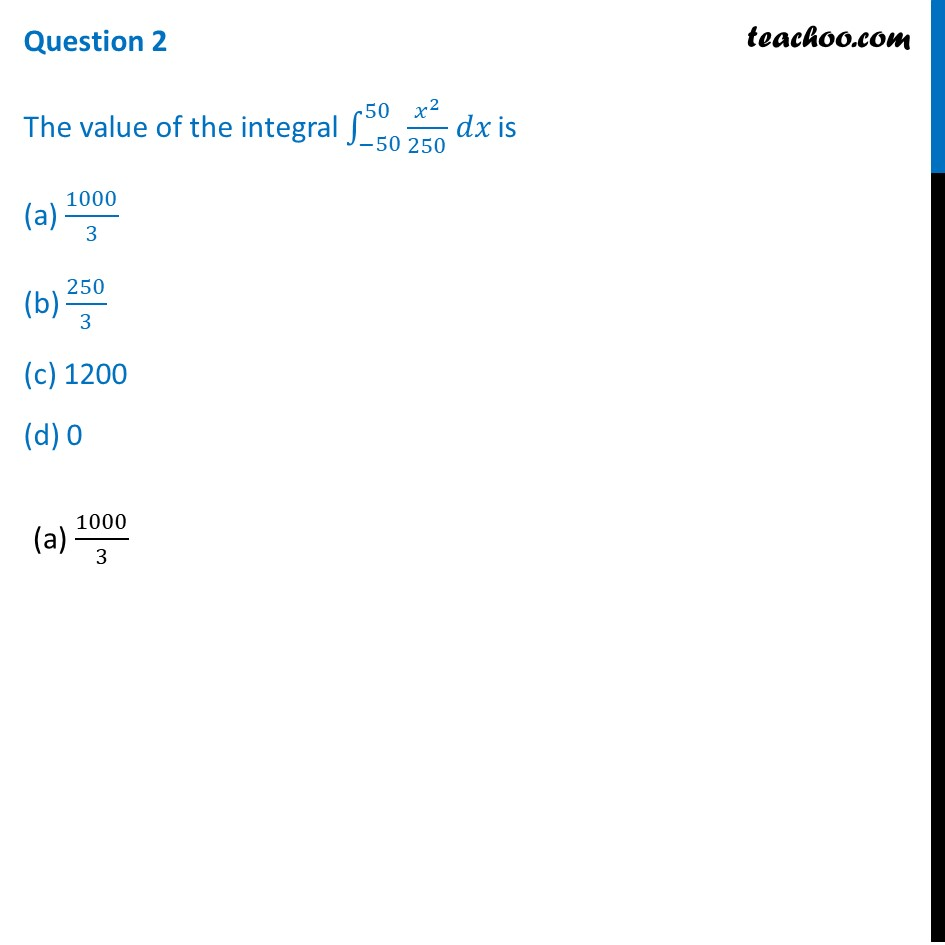## (d) None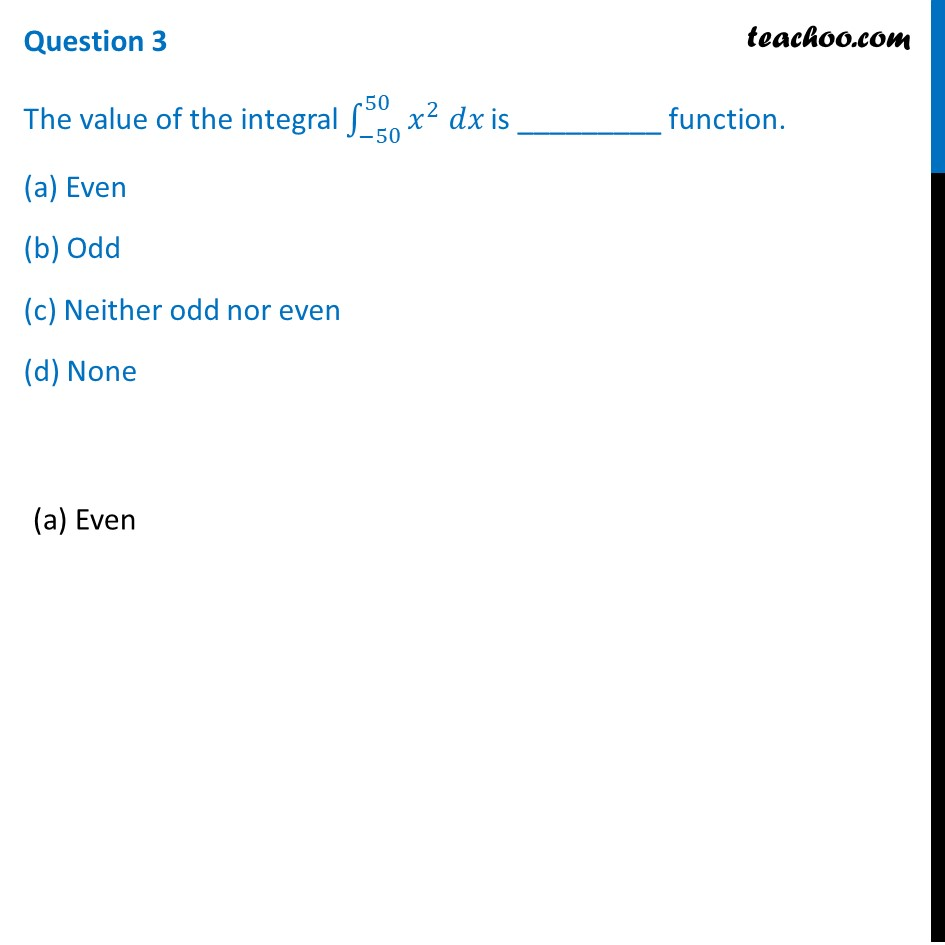## (d) 0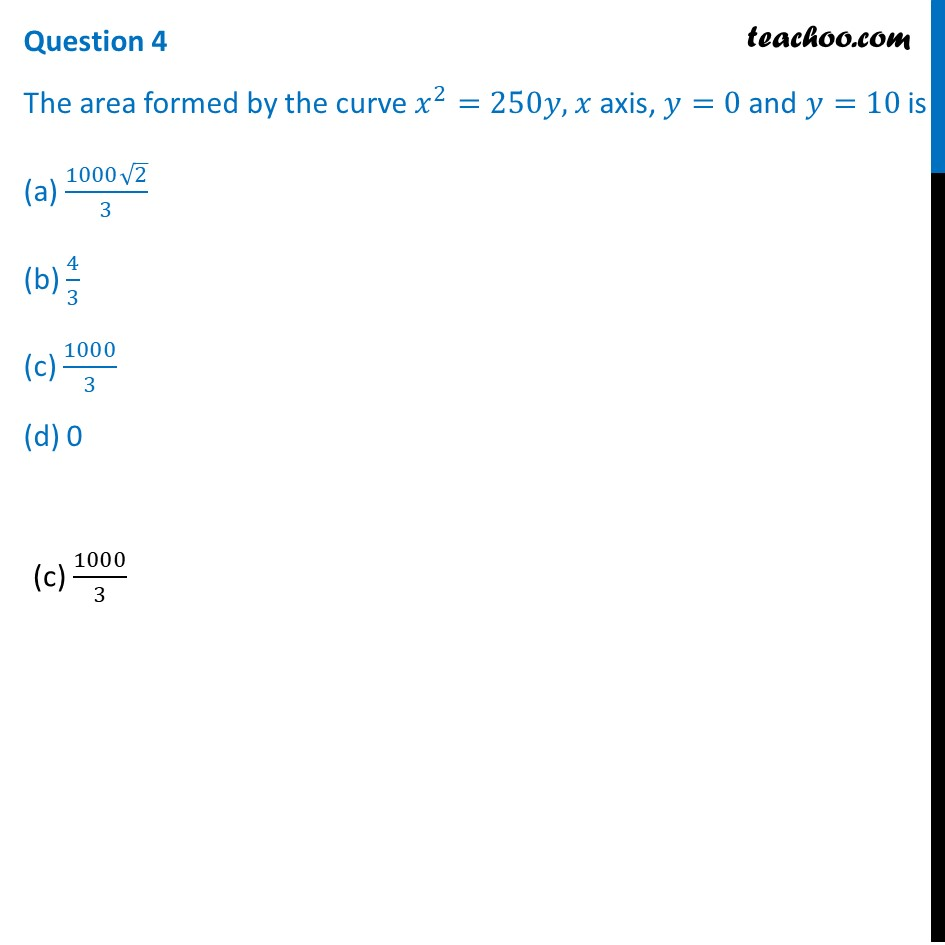## Question 5 The area formed between x 2 = 250y, y axis, y=2 and y=4 is (a) 1000/3 (b) 0 (c) (1000√2)/3 (d) none of these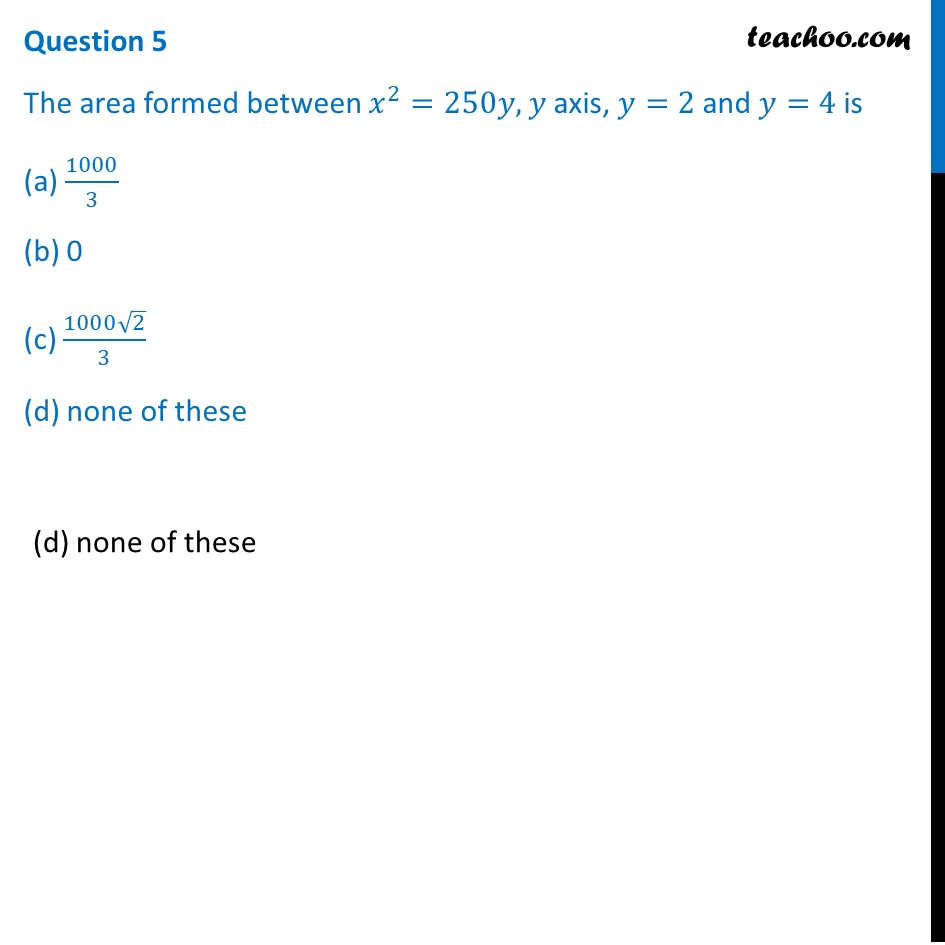Learn in your speed, with individual attention - Teachoo Maths 1-on-1 Class

### Transcript

The bridge connects two hills 100 feet apart. The arch on the bridge is in a parabolic form. The highest point on the bridge is 10 feet above the road at the middle of the bridge as seen in the figure. Based on the information given above, answer the following questions: Question 1 The equation of the parabola designed on the bridge is (a) 𝑥^2 = 250𝑦 (b) 𝑥^2 = −250𝑦 (c) 𝑦^2 = 250𝑥 (d) 𝑦^2 = 250 𝑦(b) 𝑥^2 = −250𝑦 Question 2 The value of the integral ∫1_(−50)^50▒𝑥^2/250 𝑑𝑥 is (a) 1000/3 (b) 250/3 (c) 1200 (d) 0 (a) 1000/3 Question 3 The value of the integral ∫1_(−50)^50▒𝑥^2 𝑑𝑥 is _________ function. (a) Even (b) Odd (c) Neither odd nor even (d) None(a) Even Question 4 The area formed by the curve 𝑥^2=250𝑦, 𝑥 axis, 𝑦=0 and 𝑦=10 is (a) (1000√2)/3 (b) 4/3 (c) 1000/3 (d) 0(c) 1000/3 Question 5 The area formed between 𝑥^2=250𝑦, 𝑦 axis, 𝑦=2 and 𝑦=4 is (a) 1000/3 (b) 0 (c) (1000√2)/3 (d) none of these(d) none of these